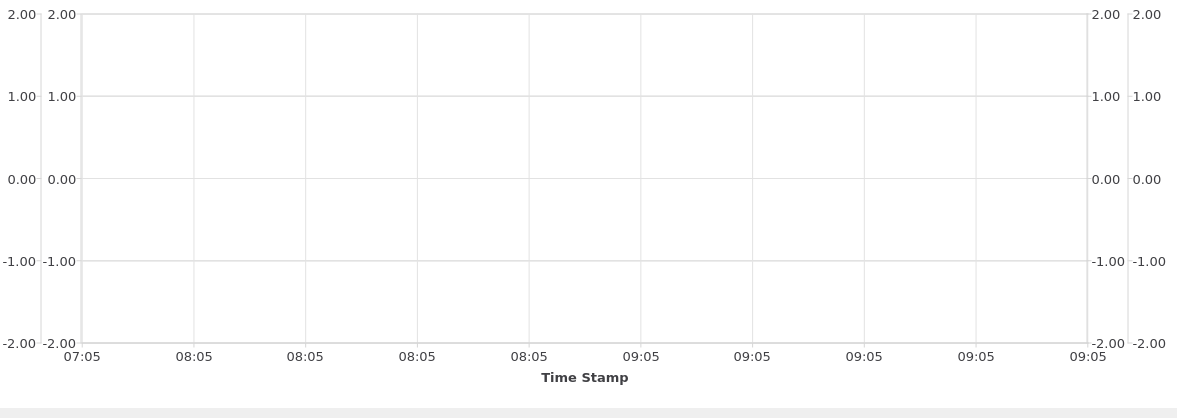# QCharts QDateTimeAxis setRange() is not working as expected.

• Hello,
I using Qlineseries for plotting the charts,
where for x axies i am using QDateTimeAxis,
and setting the min max value using QdateTime.

`````` QDateTime strtDateTime = QDateTime::currentDateTime().addSecs(-7200);
QDateTime endDateTime = QDateTime::currentDateTime();
qDebug() << "start date" << strtDateTime <<"end date" <<endDateTime;
axisX->setRange(strtDateTime, endDateTime);
``````

where debug prints is :

``````start date QDateTime(2020-05-07 07:57:58.436 UTC Qt::LocalTime) end date QDateTime(2020-05-07 09:57:58.436 UTC Qt::LocalTime)
``````

but in chart x axis taking min value as 07:50 and max value 09:05 instead of 07:57:58 and 09:57:58 respectively as attached in the screenshot, how can i set proper min and max values.

and x axies scaling is only two values even if selected 10 ticks.

Thanks
Narthan• For the chart did you call addAxis() and add the x axis to the chart? Also did you do the same thing with the line series calling attachAxis?

• @MrShawn Thanks for the response,
yes,
i have attached chart and axies.

``````series1 = new QLineSeries();

for(x;x;x) {
series1->append(epochtime, vlaue);
}

axisX = new QDateTimeAxis;

axisX->setTickCount(10);
axisX->setFormat("HH:MM");
axisX->setTitleText("Time Stamp");
QDateTime strtDateTime = QDateTime::fromString(ui->lineEdit_startDateTime->text(),"dd-MM-yyyy : hh:mm");
QDateTime endDateTime = QDateTime::fromString(ui->lineEdit_endDateTime->text(),"dd-MM-yyyy : hh:mm");
axisX->setRange(strtDateTime,endDateTime);
series1->attachAxis(axisX);
chartView->setRenderHint(QPainter::Antialiasing);
``````

• Sorry, i understand my mistake
we have to use

``````axisX->setFormat("hh : mm");
``````

instead of this i was using

``````axisX->setFormat("HH : MM");
``````